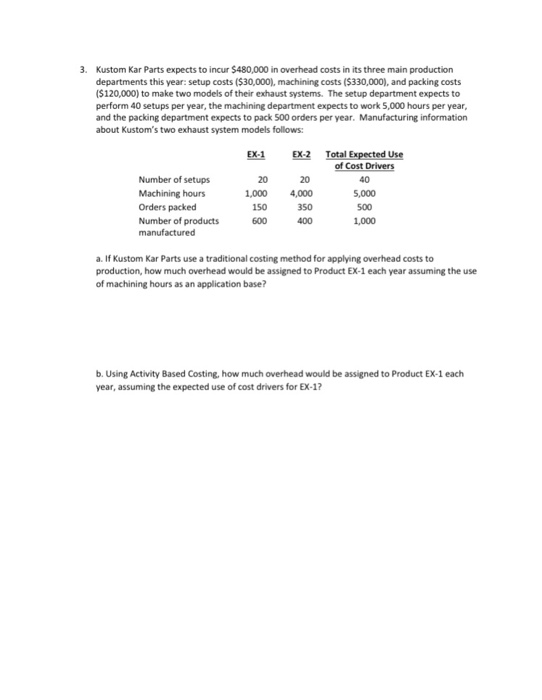# Question & Answer: Kustom Kar Parts expects to incur \$480,000 in overhead costs in its three main production departments this year: setup cost…..Kustom Kar Parts expects to incur \$480,000 in overhead costs in its three main production departments this year: setup costs (\$30,000), machining costs(\$330,000), and packing costs (\$120,000) to make two models of their exhaust systems. The setup department expects to perform 40 setups per year, the machining department expects to work 5,000 hours per year, and the packing department expects to pack 500 orders per year. Manufacturing information about Kustom’s two exhaust system models follows: a. If Kustom Kar Parts use a traditional costing method for applying overhead costs to production, how much overhead would be assigned to Product EX-1 each year assuming the use of machining hours as an application base? b. Using Activity Based Costing, how much overhead would be assigned to Product EX-1 each year, assuming the expected use of cost drivers for EX-1?

Solution:

Don't use plagiarized sources. Get Your Custom Essay on
Question & Answer: Kustom Kar Parts expects to incur \$480,000 in overhead costs in its three main production departments this year: setup cost…..
GET AN ESSAY WRITTEN FOR YOU FROM AS LOW AS \$13/PAGE

a) Under traditional costing method, Factory Overhead is calculated to allocate the factory overhead to product or job order.

– Factory Overhead Rate is the predetermined overhead rate which is used to apply manufacturing overhead to production department or products or job orders.

– Normally, it is calculated at the beginning of the period.

– It is calculated by dividing the estimated factory overhead cost by an allocation base (or suitable basis).

– Allocation bases may be direct labor hours, direct labor costs, machine hours etc.

Mathematically, FOR is calculated as follows:

Factory Overhead Rate = Estimated Manufacturing Overhead Cost / Estimated Allocation Base

Allocation base is Machine Hours in the question.

Hence,

Factory Overhead Rate = Estimated Manufacturing Overhead Cost \$480,000 / Estimated Machining Hours 5,000 Hours

= \$96 per machine hour

Assigned Overhead to Product EX-1 = Machine Hours Use for Product EX-1 1,000 hours X Factory Overhead Rate \$96 per MH

= \$96,000

b) Calculation of assigned overhead to Product EX-1 (using Activity Based Costing)

Under Activity Based Costing, overheads are calculated on the basis of activity performed in a year by the departments. We need to calculate first Cost per unit of cost driver.

Calculation of Cost Per Unit of Cost Driver

 Activities Cost/year Cost Driver Consumption of Cost Driver per year Cost per unit of Cost Driver (Cost per year / Consumption of Cost Driver Per Year) Setups \$30,000 Number of Setups 40 Setups \$750.00 Machining Costs \$330,000 Machining Hours 5000 Hours \$66.00 Packing costs \$120,000 Orders packed 500 Orders \$240.00 Total \$480,000

Calculation of Overhead Assigned to Product EX-1

 Activity Cost per unit of Cost Driver (as calculated above) Consumption of activity by Product EX-1 per year Assigned Overhead Costs to Product EX-1 Setups \$750.00 20 \$15,000 Machining Costs \$66.00 1000 \$66,000 Packing costs \$240.00 150 \$36,000 TOTAL \$117,000

Assigned Overheads to Product EX-1 using activity based costing = \$117,000